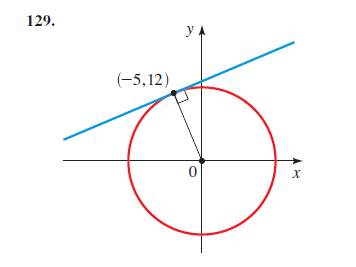# The equation for the circle and line in the given figure.### Precalculus: Mathematics for Calcu...

6th Edition
Stewart + 5 others
Publisher: Cengage Learning
ISBN: 9780840068071### Precalculus: Mathematics for Calcu...

6th Edition
Stewart + 5 others
Publisher: Cengage Learning
ISBN: 9780840068071

#### Solutions

Chapter 1, Problem 129RE
To determine

## To calculate:The equation for the circle and line in the given figure.

Expert Solution

The equation for the circle and line in the figure are x2+y2=169 and 5x12y+169=0 respectively.

### Explanation of Solution

Given information:The point P(5,12) lying on the tangent to the circle with center (h,k)=(0,0)

Formula used:

For a given circle with center (h,k) and radius r the equation is given as:

(xh)2+(yk)2=r2

This is referred to as the Standard form for the equation of a given circle.

The distance D between two points say (x1,y1) and (x2,y2) is given as:

D=(x2x1)2+(y2y1)2

Slope m of the line passing through two points in general say P1=(x1,y1) and P2=(x2,y2) is:

m=y2y1x2x1

Slope-intercept equation for a given line which has slope as m and y −intercept as b is:

y=mx+b

Two-intercept equation for a given line which has x -intercept as a and y −intercept as b is:

xa+yb=1

When two lines are perpendicular then the product of their slopes is zero that is m1.m2=1

When two lines are parallel then their slope are equal that is m1=m2

Calculation:

From the given figure it is clear that center of the circle is (h,k)=(0,0)

Recall for a given circle with center (h,k) and radius r the equation is given as:

(xh)2+(yk)2=r2

Therefore, replacing the values we get:

(x0)2+(y0)2=r2

x2+y2=r2 (1)

Now to find radius r which is the distance from the center (h,k)=(0,0) to the point P(5,12)

Recall, the distance D between two points say (x1,y1) and (x2,y2) is given as:

D=(x2x1)2+(y2y1)2

Before applying distance formula the following must be known:

x1=0y1=0x2=5y2=12

Therefore,

D=r=(50)2+(120)2=25+144=169=13

Put this value in (1)

Therefore, the required equation for circle is:

x2+y2=132x2+y2=169

Hence, the equation for the given circle is x2+y2=169

Now for the equation of line:

Recall, slope m of the line passing through two points in general say P1=(x1,y1) and P2=(x2,y2) is:

m=y2y1x2x1

As it is known:

x1=0y1=0x2=5y2=12

Therefore, slope of radius for above values is:

m1=12050m1=125

Now as the tangent is perpendicular to the circle, therefore the product of their slopes is 1

Slope of line is m2=512

And it passes through P(5,12) that is x=5y=12

Put these values in the general equation for the line which is y=m2x+b (2)

Which gives:

12=512(5)+bb=12+2512b=144+2512b=16912

Now put m2=512 and b=16912 in (2) to get:

y=512x+1691212y=5x+1695x12y+169=0

Thus, required equation of line is 5x12y+169=0

### Have a homework question?

Subscribe to bartleby learn! Ask subject matter experts 30 homework questions each month. Plus, you’ll have access to millions of step-by-step textbook answers!